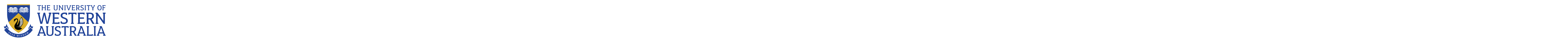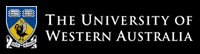School of Computer Science and Software Engineering

# School of Computer Science and Software Engineering

## CITS2211 Discrete Structures

### Lecture slides

These will be published progressively here during the course of the semester.

### Practice Questions

Practical work for this unit (tutorials) will be done during the Lecture Workshops. See below for assessment details.

### Tests and Exam

See the Asssessment page.

#### Textbook

I recommend you use the book “Mathematics for Computer Science” by Lehman, Leighton and Meyer, as the first place to turn to after the lectures. This is a 908-page behemoth that covers all we need and lots more. It is also freely available to download as a PDF, though I wouldn’t advise printing it! The direct link is: courses.csail.mit.edu/6.042/spring17/mcs.pdf

For the final part of the course, on automata and languages, I recommend the book Introduction to the Theory of Computation by Michael Sipser. This can be an expensive book, but copies are available to buy online from \$15 from e.g. www.bookfinder.com. The 3rd edition is the most recent, but earlier editions would be fine too. It is a classic text, so well worth having on your bookshelf for Computer Scientists.

It’s important to find and use a textbook that works well for you, though, and the two recommended textbooks can move quite briskly. As an alternative, you might wish to investigate some of the textbooks listed under Supplementary texts, below.

Additional suggestions for this list are welcome. Post your suggestions to help2211.

#### Logic

In addition to the recommended reading for weeks 1 through 3, you might find helpful several chapters from the “Book of Proof” by Richard Hammack. For some topics, it moves at a gentler pace than does the Lehman, Leighton and Meyer textbook (plus it also contains more sample exercises you can try). Relevant chapters are chapter 2, on propositional and predicate logic, and chapters 4 through 10, on methods of proving things.

Chapter 1 of Foundations of Computation by Carol Critchlow and David Eck covers similar material.

If you would like more practice on using truth tables – and instant, web-based feedback on whether you are right or not – you might want to try some of the online exercises from Chapter 10 of the Carnap online logic course.

Extra exercises for weeks 1-3:

• Book of Proof exercises: from sections 2.2 through 2.11. For solutions, see the Supplementary texts section below.
• Mathematical Reasoning exercises: from sections 2.1–2.2. For solutions, see the Supplementary texts section below.
• Peter Fritz exercises exercises: exercises from sections 1.8, 2.1-2.3, 2.7, 3.1-3.7, 4.1-4.2, 4.4, 7.2-7.3, 7.5, 8.1, 8.5-8.6. (Note that “$$\mathcal{L}_1$$” is just the Oxford Logic Manual’s way of saying “the language of Propositional Logic”, and “$$\mathcal{L}_2$$” is just its way of saying “the language of Predicate Logic”. So “Formalize the following English sentences in $$\mathcal{L}_2$$” means “Formalize these sentences in predicate logic.”)
• Foundations of Computation exercises: sections 1.1-1.2 and 1.4-1.5, and section 1.3 for an extension if you are intersected.
• Exercises with solutions from University of Bristol’s Writing Mathematical Proofs course: section 2, “Mathematical Logic”.

You may find useful the videos listed under the “Logic” section of the Trevtutor.com “Discrete Math 1” page, part of the Discrete Math 1 YouTube playlist. The first video is Introduction to Logic.

Other resources

Here are some additional logic resources:

• Lewis Carroll Logic puzzles
• The programming language Dafny incorporates ideas from Hoare logic. It allows programs to be verified correct while they are being developed.
• Programs which work out whether a propositional formula is satisfiable (and come up with an assignment to variables which satisfies it) are called “SAT solvers”. This presentation from Johannes Kepler University, Linz, outlines some of their practical applications.
• Unsure if you have the syntax of propositional logic formulas quite right? Type them in to this propositional formula parser to see.
• Propositional logic quizzes. For practice, try your hand at the following quiz exercises:

#### Proofs

Some classes of assertion automatically proved or disproved by computer. For example, the following websites are all capable of proving (or disproving) particular classes of statements:

Try some of these out. What sort of theorems can they prove? From lectures and the textbook, are these all theorems which you know can be mechanically proved? If not, then can you work out how the program is tackling them?

Even when a theorem cannot be automatically proved, computers can still check (or assist in the writing of) a human-produced proof. Some examples of so-called “proof assistants” are:

• Edward Z. Yang’s LogiText (source code on Github)
• ProofWeb provides online access to a range of research-level proof assistants.

Extra exercises:

You may find useful the videos listed under the “Proof Techniques” section of the Trevtutor.com “Discrete Math 1” page, part of the Discrete Math 1 YouTube playlist. The first video is Direct Proof.

Other resources:

• Unsure of the difference between a theorem, a lemma, and a corollary? Check out this Blog post on proof terminology by David Richeson.

Besides the tips in the Lehmann textbook, there are several other resources you may find useful:

• From “How To Write Proofs” (tips from Larry W. Cusick of California State University), see Part II on “Proof Strategies”
• The Book of Proof chapters 4–10.
• Two books are available which provide extensive advice on reading and writing proofs:

• D.J. Velleman, How to Prove It: A Structured Approach (2nd edn) (link to Amazon page), and
• D Solow, How to Read and Do Proofs: An Introduction to Mathematical Thought Processes (6th edn) (link to Amazon page).

Both can be obtained via the UWA Library, and bought cheaply via Amazon, Book Depository, AbeBooks, etc.

#### Sets, relations and partial orders

Extra exercises:

You may find useful the videos listed under the “Set Theory” and “Relations and Functions” sections of the Trevtutor.com “Discrete Math 1” page, part of the Discrete Math 1 YouTube playlist. The first video is Introduction to Sets.

#### Cardinality and countability

Extra exercises:

Other resources:

• "Uber ein elementare Frage der Mannigfaltigkeitslehre" This famous paper by George Cantor is the first published proof of the so-called diagonal argument, which first appeared in the journal of the German Mathematical Union (Deutsche Mathematiker-Vereinigung) (Bd. I, S. 75-78 (1890-1)). Here is the German paper with an English translation.
• Cantor's Theorem A gentle proof of Cantor's theorem.

#### Automata, languages and grammars

We look at three classes of language – regular languages, context-free languages, and Turing-recognizable languages – which are recognized by three corresponding sorts of automata (Finite State Machines, Push-Down Automata, and Turing machines, respectively).

There is another class of language we do not cover in CITS2211, the class of context-sensitive languages. Context-sensitive languages are generated by context-sensitive grammars and recognized by linear-bounded automata; context-sensitive grammars are similar to context-free grammars, but loosen the requirement that, for any production rule, there may be only one non-terminal on the left-hand side of the rule.

For an explanation of context-sensitive languages, and formal languages and automata in general, a good reference is the book Introduction to Formal Languages by György E. Révész (Dover publications, 1983, reprinted 2015, ISBN 9780486666976).

Extra exercises:

You may find useful the videos listed under the “Number Theory and Formal Languages” section of the Trevtutor.com “Discrete Math 1” page, part of the Discrete Math 1 YouTube playlist. The first relevant video is Formal Languages.

Online tools

• FSM simulator: web-page that allows you to define FSMs, display diagrams for them and simulate them being run.

Other resources

### More practice problems

• Peter Fritz’s exercises, with answers. These were designed to be used with the Oxford University Logic Manual, but the problems on sets, propositional logic and predicate logic should be soluble using the material we cover in this unit.
• The web-page for Gabriele Röger’s course on Theory of Computer Science at the University of Basel includes an “Exercises” section, with exercises and solutions in English and German.

### Supplementary, freely available texts

These may be a useful addition to the texts we follow in class.

# The University of Western Australia

## University information

CRICOS Code: 00126G